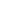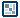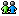## Question of the WeekScripting 3D in Flash
by senocular

Rotation Around A Common Center
This first example represents a cube rotating in 3D space but does so by rotating circle shapes around a common center all with the same rotation. Their arrangement in respect to each other make up the 8 corners of a cube shape. Despite the fact that they are just circles, the cube shape is fairly distinguishable to anyone viewing it.

[ balloon forming a rotating cube ]

Steps to Create Animation

1. Create your screen element. There's only one; a circle (or balloon). This is all that is needed for this example. No ground or anything else. In fact, it will be the last movieclip made for this collection of 3D examples. Everything beyond this will be made entirely with Actionscript. This current example revolves entirely around this one movieclip which will be attached 8 times to define the cube

2. Next is your basic scene setup creating theScene and centering it in the screen. No camera this time, but a focalLength is still needed for that scaling associated with the 3D space. Also, no objectsInScene array will be defined yet. This example will take a slightly different approach in handling the objects in the scene that will carry over better into the next examples.

1. After that, a new small function will be defined. This will create an object in Flash with 3 properties x, y and z, passed into that function. This new object, as you might have guessed, would represent a point in 3D space. The reason we are making a function to make a point like this is for the sake of ease and compactness. Instead of writing out the needed code to make an object with set x, y and z properties, this function can be easily called and that object can be created more easily for us.

1. Similarly, a 2D point making function can be made. This will act to create an object for holding just an x and a y position. However, considering how the balloon clips on the screen need to be scaled and have their depths swapped, some extra values for that can be added to this point. You'll see where this is used in the following step.

1. Now its time to get into what was effectively before, the display function(s). The idea of the display function was to provide a unique way of handling individual shapes in 3D scene each frame. That concept is going to be dropped here for something slightly different. There will still be an array of positions a la objectsInScene, only here, they will be a collection of points (created with the makePoint function) rather than movieclips. All these points, though, are each handled the same way and simply rotated without any other behaviors to separate them from the other points. The reason for this is because all those points make up the same final 3D object, a square in this example and would therefore behave the same. The balloon movieclips are then, after the 3D calculations on those points, put at the position of those points as they would exist in 2D space on the screen.

The 3D calculations on the array of points will, like before, be done in a loop. This loop, however, will be contained in a single function call which will be used to convert or transform all of the 3D points in the array into 2D points. This function will handle all trig functions and looping and produce a new array of 2D points. Before, the 2D aspect was just set to a MovieClip's _x and _y, but since the points don't necessarily have their own _x and _y, we can just make a new array to be used as the transformed screen points. The real effectiveness of this function comes in its handling of the trig functions. If you think about how the display functions work, each one of those functions when called for each movieclip in the scene re-calculated a Math.sin() and Math.cos() calculation for each movieclip given their angles and the angle of the camera. Because all of the points here behave the same and are all based the same rotation, you can greatly reduce the need for calling Math.sin() and Math.cos() speeding up processing. The truth is, you only need to call Math.sin() three times and Math.cos() three times, once for each axis of rotation and just use them for each point in the loop. Pre-calculating Math.sin(angle) and Math.cos(angle) before the loop can add some much needed speed to the 3D scene. This can be especially important later on when more complex shapes might be attempted. So this new function follows the following pattern:

1. function call:

2. pre-calculate Math.sin and Math.cos for each 3 angles of rotation

3. loop through each point in the array containing the 3D points for the scene

4. use the trig equations with the pre-calculated sine and cosine values to rotate each point in 3D space

5. based on the new x, y and z values determine a 2D x and y (along with scaleRatio and z for depth of movieclip)

6. add each of the calculated values to a new array as a 2D point

7. when done looping, return the new 2D array of 3D points transformed to 2D points

This function represents the the 3D engine for this movie. It handles all the points and rotations and transforms those points from 3D points to 2D points as they would appear on the screen. Its this function that handles most all the work and will do so for basically all of the examples that follow. Notice how temporary values are used in each of the trig functions which are then, following the operations, set to the actual final x, y and z.

1. With that 3D functionality complete you can begin mapping out the 3D points that make up your scene, in this case, the cube. This is just an array of eight 3D points made using the make3DPoint function. As you can see, the make3DPoint function makes the coordinates in for each point much more compact and easier to see and interpret being together like that. This square is 100x100x100 centered around the 3D point (0,0,0) or the center of the cube and scene.

1. Following the definition of the points, the balloons can be attached. One balloons will be attached for each point in the pointsArray. This can be done using a loop based on the pointsArray's length, attaching a balloon for each point in that array. The naming convention here will be important as it will be used later on when these clips are set to the 2D positions of each of those points after the 3D to 2D transformation - in fact, this same loop will be used again in doing that - each name being based the i value in that iteration of the loop.

1. There's one last item that needs to be created before getting to the onEnterFrame function. That's the variables for the rotations used in the cube. If you noticed in the Transform3DPointsTo2DPoints function, a passed axisRotations object was used in the sine and cosine calculations. This was an object with an x, y and z value for each rotation for that axis - and object with an x, y and z - that's just a 3D point object! The fact that there are rotations equivalent to the number of axis or values in a 3D point makes the 3D point created with the make3DPoint function a prime candidate for containing the rotations for the cube. So now, just make a 3D point to hold the rotations of the cube as it spins around in the scene.

1. Last but not least, the function to run the spinning cube - the onEnterFrame function. This performs 3 actions:
1. determines rotation values from the mouse

2. calls the Transform3DPointsTo2DPoints function to create an array of all the 3D points converted into 2D or screen points

3. and assign the position of each attached balloon to each of those new 2D points (adding in scaling and depth swapping)

Its number 3 where we see the return of the loop for the balloons. Once the 2D points have been created, we'll need to go through and correctly relate those points to each one of those attached clips. Since they were created based on the pointsArray, they can be assigned the same way. The difference being you are assigning based on the new array of 2D points and not the original 3D points in pointsArray. That, however, doesn't matter since the new array of points is the same as pointsArray, just in 2D terms and not 3D. With that assignment, the 3D cube is fully functional.

Note that the mouse only changes the x and y values in cubeAxisRotations. The z axis isn't even changed, though it still seems as if (and really is) a full range of 3D rotation is achieved.SUGGESTION: Avoid Re-looping
 This last example with the rotating cube with the balloons loops through the pointsArray 2 times, once to transform the points and create the array of 2D points and another to cycle through and assign each movieclip to its corresponding screen position. Looping twice like inefficient and could slow-down your movie. It would be best to perform all the actions in one loop. However, that example was to setup the new Transform3DPointsTo2DPoints function which will be used in the examples to follow that don't have the extra loop since there are no attached movieclips involved. The only loop will be the one in Transform3DPointsTo2DPoints.

The 3D Engine
The Transform3DPointsTo2DPoints function of this past example is the grounds from which all following examples will be based. It will be the engine running each of those movies. There may be some changes here and there, but the general functionality will remain the same. As an engine, Transform3DPointsTo2DPoints is actually quite simple only performing the basics of point transformation and doesn't even handle other operations such as the displaying of visuals etc. for you which other, and probably most, 3D engines would. That process, however, will be done manually and slightly different concerning in the remaining examples so it's not incorporated into the function as it exists now. You are free to do so on your own, though. There is no set way of doing things and other 3D engines for Flash could be completely different than this one. This is just one way of getting the job done.

The Drawing API
The drawing functions in Flash was only just touched upon in the first example. Its about time we started pushing what they have to offer in terms of getting a true rendered 3D shape out of all that has been learned so far in creating a 3D scene in Flash. No attached movieclips involved here.